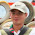-->

## 2007/06/18

### 態度決定你的一切

A=1, B=2, C=3, D=4, E=5 ..... Z=26

knowledge = 11 + 14 + 15 + 23 + 12 + 5 + 4 + 7 + 5 = 96

hardwork = 8 + 1 + 18 + 4 + 23 + 15 + 18 + 11 = 98

attitude = 1 + 20 + 20 + 9 + 20 + 21 + 4 + 5 = 100

#### 3 則留言:

1.OGCOGCOGCOGC可以得到幾分

2.分數破表，不然就是查不到這個單字 XD

3.以前我是從事 Human Resource,
大企業通常每一個職位都設有職位說明書,